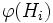# Homomorph-containment is strongly join-closed

This article gives the statement, and possibly proof, of a subgroup property (i.e., homomorph-containing subgroup) satisfying a subgroup metaproperty (i.e., strongly join-closed subgroup property)
View all subgroup metaproperty satisfactions | View all subgroup metaproperty dissatisfactions |Get help on looking up metaproperty (dis)satisfactions for subgroup properties
Get more facts about homomorph-containing subgroup |Get facts that use property satisfaction of homomorph-containing subgroup | Get facts that use property satisfaction of homomorph-containing subgroup|Get more facts about strongly join-closed subgroup property

## Statement

### Statement with symbols

Suppose$G$ is a group,$I$ is an indexing set, and$H_i, i \in I$, is a collection of homomorph-containing subgroups of$G$. Then, the join of subgroups$\langle H_i \rangle_{i \in I}$ is also a homomorph-containing subgroup of$G$.

## Related facts

Given: A group$G$, an indexing set$I$, a collection$H_i$ of homomorph-containing subgroups of$G$,$i \in I$.$H = \langle H_i \rangle_{i \in I}$. A homomorphism$\varphi: H \to G$.
To prove:$\varphi(H)$ is containined in$H$.
Proof:$\varphi(H) = \varphi \langle H_i \rangle_{i \in I} = \langle \varphi(H_i) \rangle_{i \in I}$. Since each$H_i$ is homomorph-containing in$G$,$\varphi(H_i)$ is contained in$H_i$, so the join of$\varphi(H_i), i \in I$, is contained in the join of the$H_i$s, which is$H$.